# AP Physics B : Electricity

## Example Questions

### Example Question #1 : Electricity

Charges A and B are placed a distance of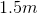from one another. The charge of particle A is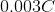whereas the charge of particle B is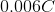. Charge B experiences an electrostatic force of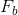from charge A. Similarly, charge A experiences an electrostatic force of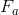from charge B.

A student places these two charges in a vacuum medium and measures. A second student places these two charges in a glass medium and measures. What is ratio of the force measured in the glass medium to the force measured in the vacuum?

The dielectric constant of glass is.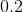Explanation:

You can simplify this question tremendously by using the definition of a dielectric constant. Dielectric constant is defined as the ratio of the electrostatic force in vacuum to the electrostatic force in the medium (in this case glass).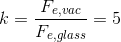The question is asking for the reciprocal of this value: the ratio of the force in glass to the force in the vacuum. Our answer is calculated by taking the reciprocal of the dielectric constant of glass.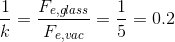### Example Question #1 : Electricity And Magnetism

How much work is done by the electric field moving an electron along an equipotential surface with a potential of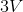?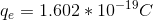We must know the strength of the electric field to solve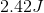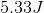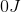Explanation:

Moving a charge along an equipotential surface will involve no work done by the electric field. The potential is constant throughout an equipotential surface; therefore, the potential difference experienced by the electron will be zero. Remember that energy is dependent on the potential difference.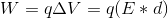If the potential difference is zero then the energy (and work) will be zero.

### Example Question #1 : Coulomb's Law

Charges A and B are placed a distance offrom one another. The charge of particle A iswhereas the charge of particle B is. Charge B experiences an electrostatic force offrom charge A. Similarly, charge A experiences an electrostatic force offrom charge B.

What is the ratio ofto?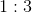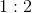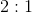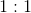Explanation:

This question is very simple if you realize that the force experienced by both charges is equal.

The definition of the two electrostatic forces are given by Coulomb's law: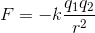In this question, we can rewrite this equation in terms of our given system.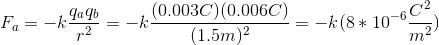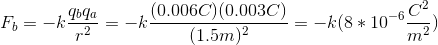It doesn’t matter if the charges of the two particles are different; both particles experience the same force because the charges of both particles are accounted for in the electrostatic force equation (Coulomb's law). This conclusion can also be made by considering Newton's third law: the force of the first particle on the second will be equal and opposite the force of the second particle on the first.

Since the forces are equal, their ratio will be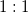.

### Example Question #1 : Coulomb's Law

An excess charge of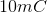is put on an ideal neutral conducting sphere with radius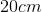. What is the Coulomb force this excess charge exerts on a point charge of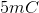that is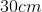from the surface of the sphere?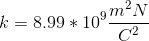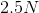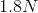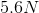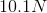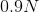Explanation:

Two principal realizations help with solving this problem, both derived from Gauss’ law for electricity:

1) The excess charge on an ideal conducting sphere is uniformly distributed over its surface

2) A uniform shell of charge acts, in terms of electric force, as if all the charge were contained in a point charge at the sphere’s center

With these realizations, an application of Coulomb’s law answers the question. If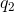is the point charge outside the sphere, then the force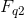onis: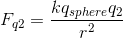In this equation,is Coulomb’s constant,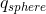is the excess charge on the spherical conductor, andis total distance in meters offrom the center of the conducting sphere.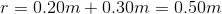Using the given values in this equation, we can calculate the generated force: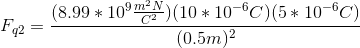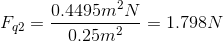### All AP Physics B Resources